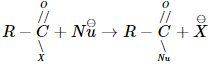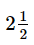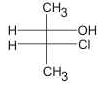Courses

# VITEEE Chemistry Test - 7

## 40 Questions MCQ Test VITEEE: Subject Wise and Full Length MOCK Tests | VITEEE Chemistry Test - 7

Description
This mock test of VITEEE Chemistry Test - 7 for JEE helps you for every JEE entrance exam. This contains 40 Multiple Choice Questions for JEE VITEEE Chemistry Test - 7 (mcq) to study with solutions a complete question bank. The solved questions answers in this VITEEE Chemistry Test - 7 quiz give you a good mix of easy questions and tough questions. JEE students definitely take this VITEEE Chemistry Test - 7 exercise for a better result in the exam. You can find other VITEEE Chemistry Test - 7 extra questions, long questions & short questions for JEE on EduRev as well by searching above.
QUESTION: 1

Solution:
QUESTION: 2

Solution:
QUESTION: 3

### What is the product obtained when chlorine reacts with ethyl alcohol in the presence of NaOH?

Solution:
QUESTION: 4

Propyne on hydrolysis in presence of HCl and HgSO₄ gives

Solution:
QUESTION: 5

Octance number is zero of

Solution:
QUESTION: 6

Which species represents the electrophile in aromatic nitration?

Solution:
QUESTION: 7
Which alkene gives the same product by the Markownikoff's and anti-Markownikoff's method
Solution:
QUESTION: 8

Heterolysis of carbon-chlorine bond produces

Solution:
QUESTION: 9

DNA multiplication is called

Solution:
QUESTION: 10

On heating glucose with Fehling's solution we get a precipitate whose colour is

Solution:
QUESTION: 11

Peptisation denotes

Solution:
QUESTION: 12

Amides may be converted into amines by a reaction named after

Solution:
QUESTION: 13

[Ag(NH₃)₂]OH liberates silver when it reacts with

Solution:
QUESTION: 14

The reaction,is fastest when X is

Solution:
QUESTION: 15

Just before attaining equilibrium by a reversible reaction, it is found that

Solution:
QUESTION: 16

In a chemical equilibrium Kc = Kp when :

Solution: Kp = Kc when Δn = 0
QUESTION: 17

Given that
2C(s)   + 2O2g → 2CO2(g)    , ΔH = − 787 k J
H2(g)    + 1/2O2g → H2O(l)    , ΔH = − 286 k J
C2H2(g) +O2g → 2CO2+ H2Ol , ΔH = − 1301 k J
Heat of formation of acetylene is

Solution:
QUESTION: 18

Which of the following reaction is endothermic?

Solution:
QUESTION: 19

In thermodynamics, a process is called reversible when

Solution:
QUESTION: 20

The dyes attached to the fibres themselves by irreversible chemical reactions are

Solution:
QUESTION: 21

CH₃CH₂CH₂CH(CH=CH₂)CH₂CH₂CH₃ is

Solution:
QUESTION: 22

In Fe(CO)₅ the Fe-C bond possesses

Solution:
QUESTION: 23

The IUPAC name of K₃[Ir(C₂O₄)₃] is

Solution:
QUESTION: 24

In the diazotisation of aniline the excess of hydrochloric acid is used primarily with sodium nitrite because it

Solution:
QUESTION: 25

phenyl isocyanides are prepared from which of the following reactions ?

Solution:
QUESTION: 26

Oxidation number of S in H₂S₂O₈ is

Solution:
QUESTION: 27

Strong electrolytes are those which

Solution:
QUESTION: 28

When 2.5 amp. of current passed through CuSO4 solution for 6 min. 26 sec. the amount of copper deposited is

Solution:
QUESTION: 29

Anisole with HNO₃ and conc. H₂SO₄ gives

Solution:
QUESTION: 30

The slag obtained during the extraction of copper from pyrites is composed mainly of

Solution:
QUESTION: 31

A container contains 1 mole of a gas at 1 atm pressure and 27oC, and its volume is 24.6 ltres. If pressure is 10 atm and temperature 327oC, then new volume is approximately

Solution:
QUESTION: 32

Which of the following halides is the most stable?

Solution:
QUESTION: 33

Metaphosphoric acid has the formula

Solution:
QUESTION: 34

The largest bond angle is in

Solution:
QUESTION: 35
Which of the following is hcp crystal structure?
Solution:
QUESTION: 36

In the reaction 4Fe + 3O₂ → 4Fe3+ + 6O₂- which of the following statement is incorrect ?

Solution:
QUESTION: 37

Compound A belowSolution:
QUESTION: 38

Adsorbed acetic acid on activated charcoal is

Solution:
QUESTION: 39

Transition elements are

Solution:
QUESTION: 40

The number of ions formed on dissolving one molecule of FeSO₄ (NH₄)₂SO₄. 6H₂O is/are

Solution: